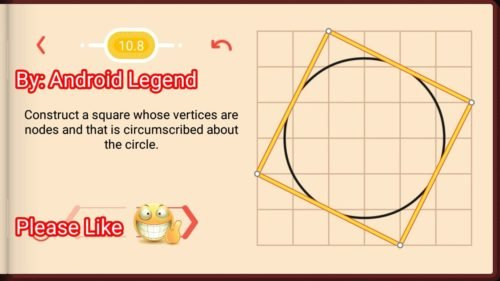# Pythagorea Level 10.1 10.2 10.3 10.4 10.5 10.6 10.7 10.8 Solution/AnswersPythagorea is android/iOS app developed by Horis International Limited. Solutions hints and answers to pythagorea are available in this post scroll down to find solutions to all the levels.

This game is mostly focused on geometric puzzles and construction. the workspace is divided into grids to draw lines. You should know all the basic Math operations. All lines and shapes are drawn on a grid whose cells are squares. Most of the game levels can be answered using natural intuition and bye some basic laws of geometry.

You have to connect points on the grid using straight lines to construct an element, you can even use intersection points to draw. some levels are very easy some are of medium difficulty and some are very hard to solve, that’s why I am providing solutions to all the problems.

If you have forgotten basic course you did in your elementary education, this game is for you to revise all the concept using a single game or if you don’t know any of the geometry this game features “i” button from where you can learn about all the shapes and geometry and then play this game to enhance your geometry skill.And do not miss your chance to familiarize children with mathematics. Pythagorea is an excellent way to make friends with geometry and benefit from spending time together.

If you are here for levels other than ‘Circles’ Go to directory of all other levels at : http://www.puzzlegamemaster.com/pythagorea-all-answers-solutions/

Pythagorea Level 10.1: Construct the center of the circle.Pythagorea Level 10.2: Construct a diameter of the circle through the point A.Pythagorea Level 10.3: Construct the center of the circle.Pythagorea Level 10.4: Construct a square whose vertices are nodes and that is circumscribed about the circle.Pythagorea Level 10.5: Construct a square whose vertices are nodes and that is circumscribed about the circle.Pythagorea Level 10.6: Inscribe a square in the circle using the point A as its vertex.Pythagorea Level 10.7: Inscribe a square in the circle using the point A as its vertex.Pythagorea Level 10.8: Construct a square whose vertices are nodes and that is circumscribed about the circle.Feel free to comment below if you have any doubts regarding the solutions. I will try to help you guys. All other levels are posted on this blog please visit them too.